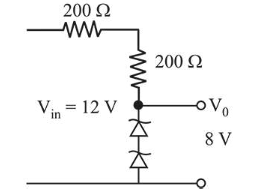# The circuit shown below is working as a 8 V dc regulated voltage source.

Question:

The circuit shown below is working as a $8 \mathrm{~V}$ dc regulated voltage source. When $12 \mathrm{~V}$ is used as input, the power dissipated (in $\mathrm{mW}$ ) in each diode is; (considering both zener diodes are identical)___________Solution:

(40) Current in the circuit, $I=\frac{12-8}{400}=10^{-2} \mathrm{~A}$

Power dissipited in each diode, $P=V I$

$\Rightarrow P=4 \times 10^{-2}=40 \mathrm{~mW}$